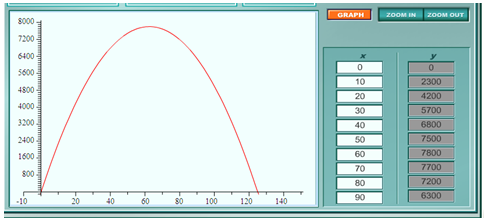Revenue Problems

Suppose the revenue earned from the sale of bicycles at the City Bike Shop is given as R(x) = 250x - 2x2. Here x = the price of a bike and R is the revenue earned. The graph can help determine the maximum revenue that can be earned as well as the price that will provide the most revenue.

Example Use the table and graph below (created from the equation above) to determine the price that will provide the maximum revenue for the bike shop.Step 1. Using a graphing calculator, find the vertex.

The vertex is (62.5, 7812.5).

Step 2. Use the vertex to answer the question.

Since the graph opens down, the vertex will be the maximum value. This is the point where the price will yield the maximum revenue. This means the maximum revenue is \$7,812.50 and the price that will produce this maximum revenue is \$62.50 per bike.